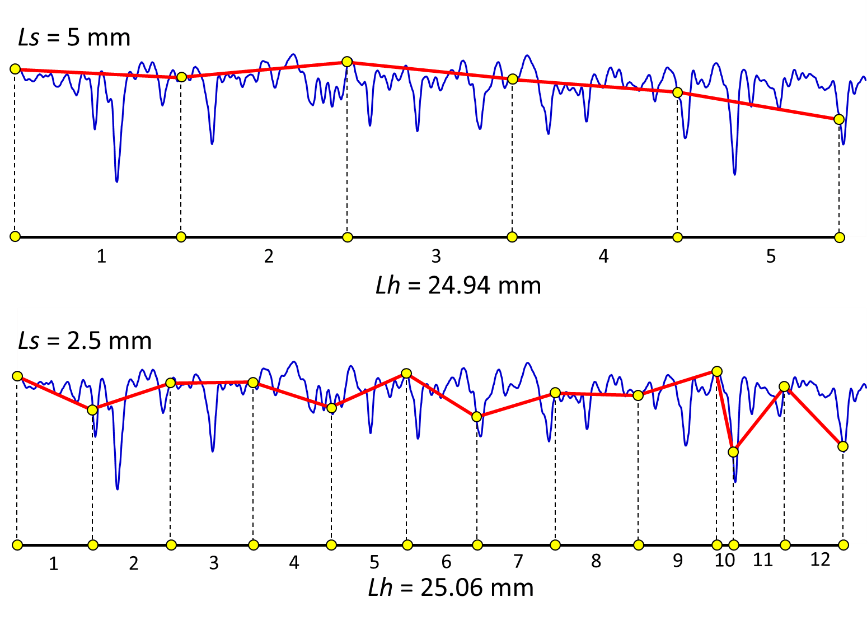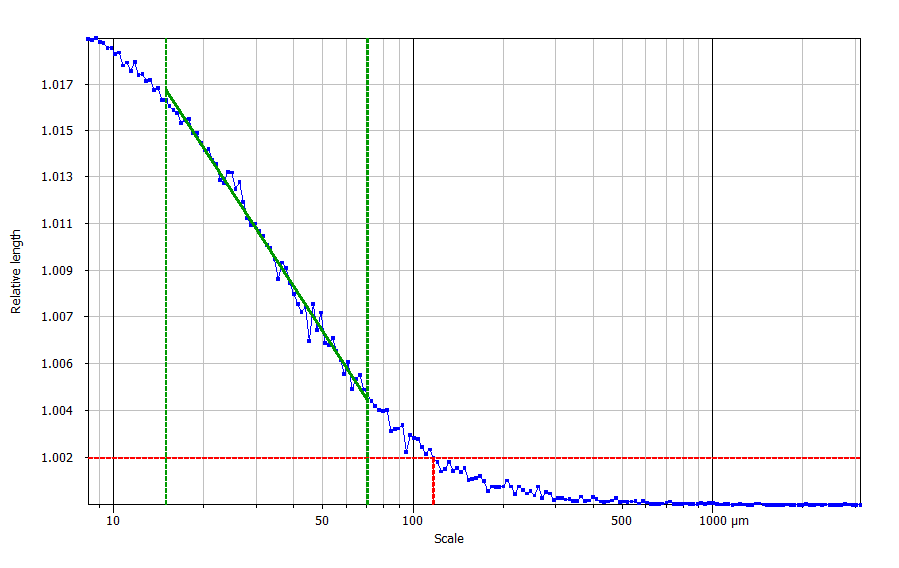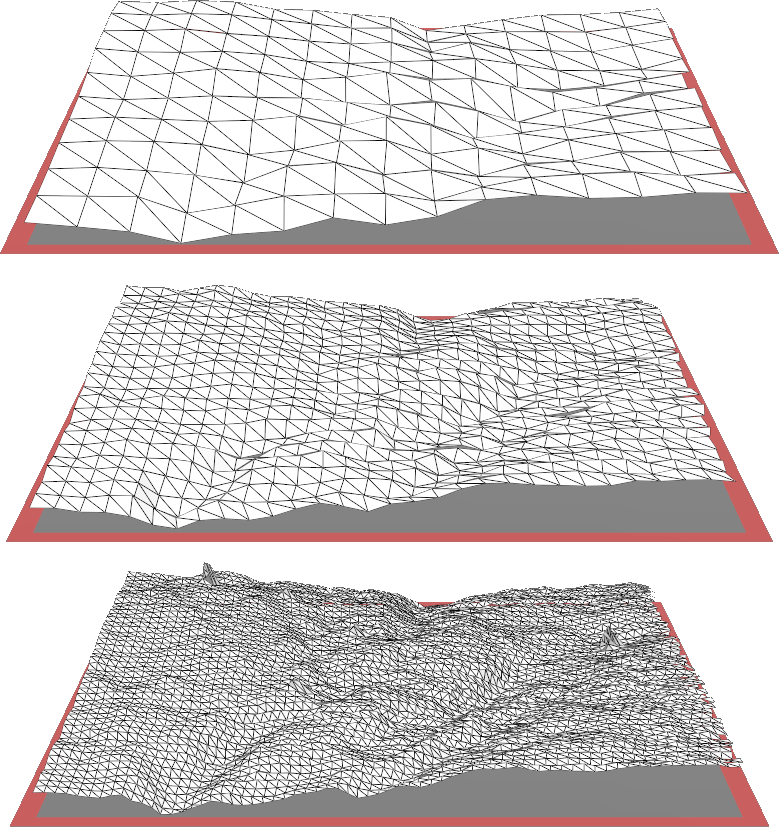# Using Scale-Sensitive Fractal Analysis: Five Things to Consider

The surface metrology expert at Digital Surf, François Blateyron, outlines what Scale-Sensitive Fractal Analysis is and how it is used, as a new SSFA optional module is made available to users of Mountains® software.

## What is Fractal Analysis?

The fact that geometrical objects are sorted by the number of coordinates needed to describe them, is something we were all taught in school. Cubes are 3-dimensional, whereas planes are 2-dimensional and a line segment constitutes the first dimension. Yet, in reality, lines can become much more complex.American-French-Polish mathematician Benoit Mandelbrot outlined the foundations of a new branch of mathematics which treated geometrical dimensions as fractional rather than integral. With several fascinating applications in fields as diverse as physics, economy and the arts, this theory outlines a fractal dimension which proved an intriguing parameter by which physical surfaces – and particularly engineering surfaces – could be described.

For rough surfaces, which are like microscopic mountainous surfaces, it is possible to understand their fractal dimension as an attribute which characterizes their complexity. While the fractal dimension of extremely smooth and planar surfaces tends to be close to 2.0, the multiple pores and cavities of ceramic surfaces produce a higher value which is nearer to 3.0.

The application of Mandelbrot’s work to engineering surfaces was advanced particularly by Christopher Brown – a Professor at the Worcester Polytechnic Institute (WPI). Along with various other parameters and statistical methods, he introduced analyses of length-scales and area-scales in order to demonstrate both functional correlations and discriminations.

Scale-sensitive fractal analysis (SSFA) is the term used to describe these techniques.

## Scale-Sensitive Graphs

During length-scale analysis, a profile’s (or surface line’s) actual length is measured via the use of a line-segment of fixed length, known as the reference scale. Relative length is determined by dividing the cumulated length by the horizontal length, producing a value between 1.0 and 2.0.In this first example, a profile is measured with the use of a 5 mm long segment. Five segments fit into a horizontal length of 24.94 mm. Consequently, the relative length is defined as 25.0/24.94 = 1.0024.

When using a segment of 2.5 mm, however, a horizontal length of 25.06 mm can accommodate 12 segments. This produces a relative length equaling 1.197. To put it another way, as the segment gets smaller, it yields a longer measured length and a higher relative length.

Different segment lengths are used to repeat the measure and produce a graph of log(relative length) in function of log(scale).A threshold is set on this graph in order to locate the smooth-rough crossover (SRC). This gives the scale of transition between large scales – in which a surface has a fractal dimension near to the Euclidian dimension 1.0 as it is analyzed from too far away - and scales where the behavior of the surface really is described by the fractal dimension. Between the green lines, the central area is nearly linear. The fractal dimension can be calculated using the slope in this section.

## Can the Same Method be used to Analyze a Surface?

Yes it can, as lines or columns of a surface can be used to calculate relative length. The relative length’s average value can then be used to build the graph.

An alternative option is the use of area-scale method. In this process, the actual area of a surface is calculated using triangular tiles of a fixed area. The actual area is then divided by the projected area to give the relative area. This produces a similar graph which can be used to calculate the same parameters.Three tiling exercises with different tile sizes.

## Related Parameters

Several different fields of metrology and research utilize these parameters. As well as others, these fields include fracture analysis, evaluating adhesion strength, thermal-spray coating and tool wear.

The parameters listed below are described in ISO 25178-2, clause 4.4.9. They are also part of ASME B46.1:2002.

• Smooth-rough crossover: a particularly useful parameter for friction and contact related applications.
• Maximum relative length or area: on a given surface, this value – taken at the finest scale - converges towards the ISO parameter Sdr.
• Fractal dimension: this is calculated using the linear part of the graph.
• Complexity: expresses how much more complex the surface is than a Euclidian plane, or a profile from a Euclidean line. This concept is reasonably comparable to the ISO parameter Sdq.
• Scale of maximum complexity: this parameter is used in correlation studies with functional attributes, and is defined as the scale at which the surface exhibits a higher complexity.
• Regression coefficient R²: the accuracy of the complexity value is confirmed by a high value.
• Heterogeneity of complexity: calculates the dispersion of complexity and analyzes the surface by blocks such as ‘5x5’.

The above parameters are calculated within a scale range in which the function is found to be active. Using a scale-limited approach is advantageous as compared with a default filter, as the former method is a more powerful means of calculating a field parameter on a surface than the latter.

## Where can I Find the Tools?

A new optional module named Scale-Sensitive Fractal Analysis is offered on the latest versions of MountainsMap® 7.4. SSFA implements the majority of the methods which were available in Professor Brown’s Sfrax software. There is a free 30 day trial of this module available. Alternatively, it can be purchased as a separate module.This information has been sourced, reviewed and adapted from materials provided by Digital Surf.

## Citations

• APA

Digital Surf. (2019, December 24). Using Scale-Sensitive Fractal Analysis: Five Things to Consider. AZoNano. Retrieved on July 08, 2020 from https://www.azonano.com/article.aspx?ArticleID=4990.

• MLA

Digital Surf. "Using Scale-Sensitive Fractal Analysis: Five Things to Consider". AZoNano. 08 July 2020. <https://www.azonano.com/article.aspx?ArticleID=4990>.

• Chicago

Digital Surf. "Using Scale-Sensitive Fractal Analysis: Five Things to Consider". AZoNano. https://www.azonano.com/article.aspx?ArticleID=4990. (accessed July 08, 2020).

• Harvard

Digital Surf. 2019. Using Scale-Sensitive Fractal Analysis: Five Things to Consider. AZoNano, viewed 08 July 2020, https://www.azonano.com/article.aspx?ArticleID=4990.